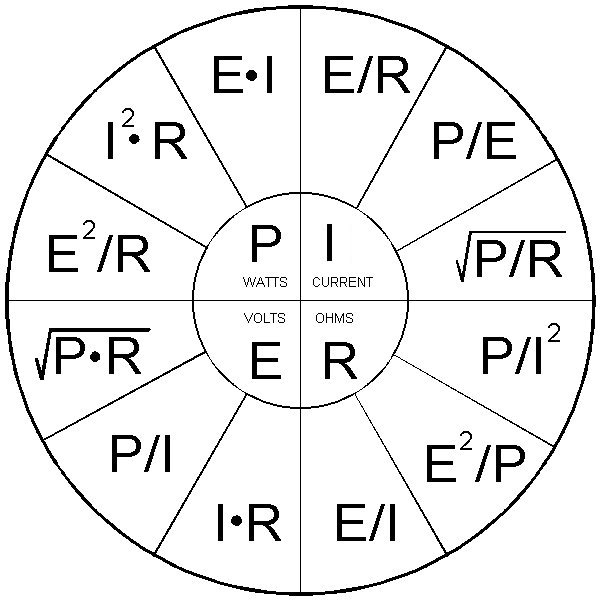# Need help with some math. Building a low pass filter

Discussion in 'Home Brew' started by loosecannon, Oct 15, 2019.

1. ### loosecannon Sr. Member

Joined:
Mar 9, 2006
Messages:
3,608
2,299
Hello all,

I am looking for some help with filter design.

Not necessarily how to figure the parts values as there are plenty of online calcs for that.

What im looking for is how to figure out the voltage rating of the shunt capacitors.

for example, if i wanted my low pass filter to be rated to handle 2000 watts continuous, what voltage rating of capacitors do i need to use?

Thanks in advance for any help on this.
LC

#1

2. ### Captain Kilowatt Professional Amateur Staff Member

Joined:
Apr 6, 2005
Messages:
15,761
8,096
Same basic Ohms Law calculations. 2000 watts into 50 ohms is about 325 volts of RF. Factor in a margin for high SWR etc. 500 volt caps would be the minimum I would use and you should be safe with those.

#2
binrat and loosecannon like this.
3. ### 543_Dallas Sr. Member

Joined:
Jul 25, 2011
Messages:
2,177
2,466
The formula I've been using came from an AG6K article. The exponent didn't copy and paste right. 20.5 is actually 2 to the .5 power. http://www.somis.org/bbat.html

"The RF-peak-voltage that appears across the capacitor varies widely depending on the feed-line Z and the power level used. The voltage can be calculated if the impedance and power at the feed-point are known. The formula for calculating this is E= [P x Z]0.5 . Since P is measured in RMS-watts, the AC voltage (E) result will be in RMS-volts which can be converted to peak-volts (e) by multiplying the result by 20.5 . For example, if Z=600ohm and P=1500W, e= [1500W x 600ohm] *20.5 =1341v peak.

When using a vacuum variable-capacitor, it is important to realize that the maximum RF peak-voltage rating at 30MHz is usually 60% of the rated DC / 60Hz peak-voltage rating. Thus, a "5000V" rating translates to a 3000 peak RF-volt rating."

#3
4. ### Captain Kilowatt Professional Amateur Staff Member

Joined:
Apr 6, 2005
Messages:
15,761
8,096

This is true. I just gave him the numbers for 50 ohms since I assumed that was what he was looking for. In any event the LPF would have to be designed for the impedance of the system hence my assumption of 50 ohms.

#4
5. ### loosecannon Sr. Member

Joined:
Mar 9, 2006
Messages:
3,608
2,299
thanks to both of you for the answers.

looks like RMS is 316.23 volts, and peak is 447.21.

Im just going to go with 1kv caps then because why not.

Yes, i could have gone to google and found the equation myself, but i like to use this forum for the great resource that it is, and in case someone in the future has a similar question.
LC

#5
6. ### 543_Dallas Sr. Member

Joined:
Jul 25, 2011
Messages:
2,177
2,466
Another useful formula that I picked up from a guy. Rf current can be figured by amps x amps x impedance. 800 watts into 50 ohms would be 4 amps. 4x4x50=800. This is 800w carrier no pee e pee.

There is a way to work it the other way if you don't know current but I'm not a math wiz. I plug numbers in until I get the wattage I'm looking for. Use larger conductors than you would for DC. Think skin effect.

#6
loosecannon likes this.
7. ### Captain Kilowatt Professional Amateur Staff Member

Joined:
Apr 6, 2005
Messages:
15,761
8,096
You can find one thing if you know the other two.#7
L2 and binrat like this.
8. ### loosecannon Sr. Member

Joined:
Mar 9, 2006
Messages:
3,608
2,299
I've got one of those ohms wheels up on my bulletin board, but i assumed that there was something more complicated going on since i am dealing with RF voltage.

using ohms law gives you 325 volts, and using the formula that 543 linked to gives
316 volts RMS and 447 volts peak.

CK, are you saying that the other formula is not needed and i can just use ohms law when working with RF voltages?
trying to learn a bit here.
LC

#8
9. ### Captain Kilowatt Professional Amateur Staff Member

Joined:
Apr 6, 2005
Messages:
15,761
8,096

I rounded to 325. My numbers are RMS. 325 volts is actually 2112.5 watts into 50 ohms. Exactly 2000 watts is 1997.12 watts.

#9
loosecannon likes this.
10. ### 500kpot New Member

Joined:
Jan 17, 2019
Messages:
29
9
I think the formula referenced above
Should be expressed as ((Watts x Resistance)*2)^.5
To the power of .5 is the same as square root.

The expression (1500x600) * 2^5 does not equal 1314.
1500x600 = 900000
2^.5 =1.414
90000 x 1.414 = 1272600

On the other hand
1500 x 600 = 900000
900000 x 2 = 1800000
1800000^.5 = 1314

I hope that helps you figure out what caps you need!

And to Mr 543 - Thank you for posting that link. Man, lots of great information. I really enjoy learning from others on this forum and I hope I can contribute even just a bit.

#10